# Selina Solutions Concise Maths Class 10 Chapter 8 Remainder and Factor Theorems Exercise 8(C)

Problems based on both factor theorem and remainder theorem are included in this exercise. If students wish to get a strong grip on this chapter, the Selina Solutions for Class 10 Maths is the best place to start with. All the solutions are prepared in a simple language to make it easier for students to understand. For solutions to the Concise Selina Solutions for Class 10 Maths Chapter 8 Remainder and Factor Theorems Exercise 8(C), the PDF is available in the links given below.

## Selina Solutions Concise Maths Class 10 Chapter 8 Remainder and Factor Theorems Exercise 8(C) Download PDF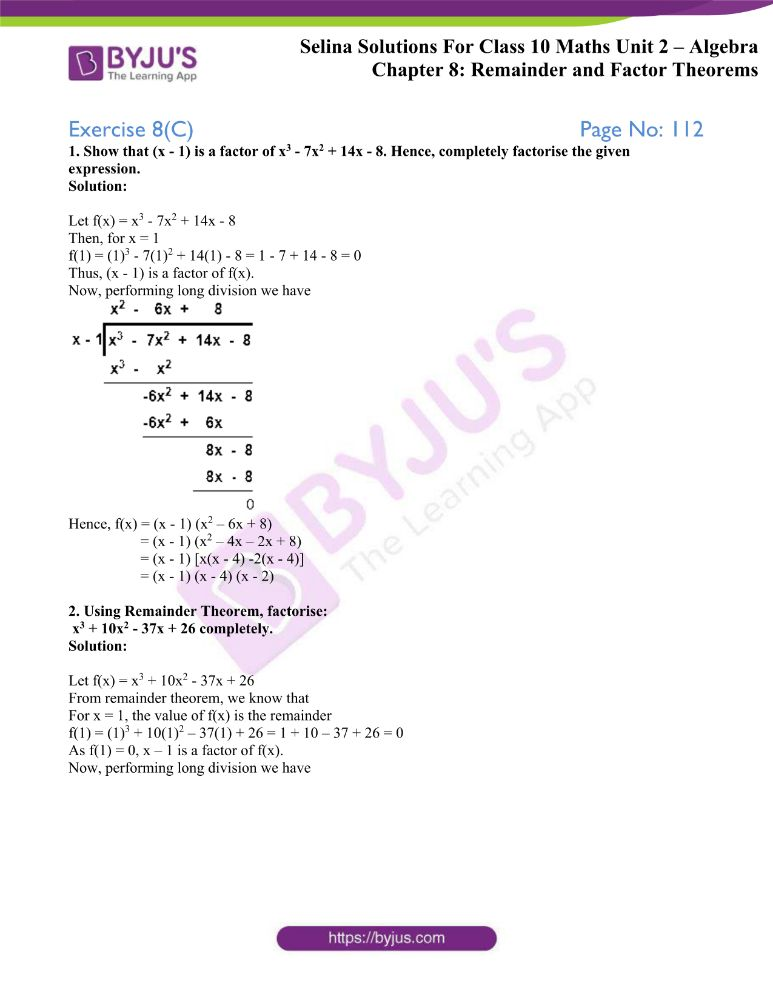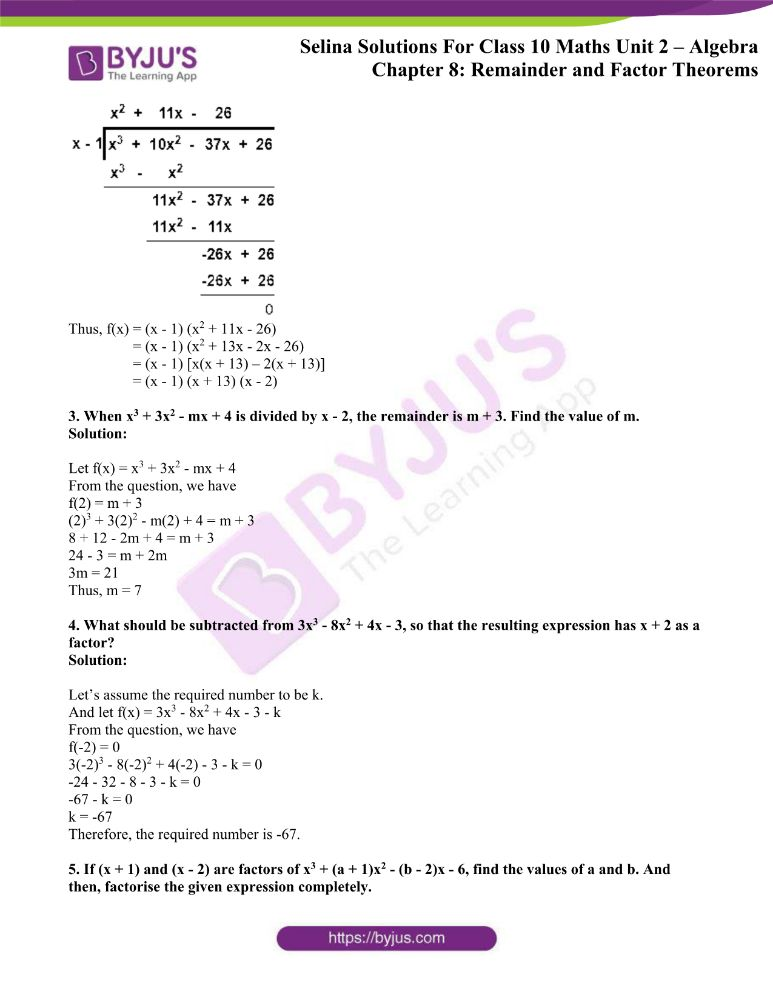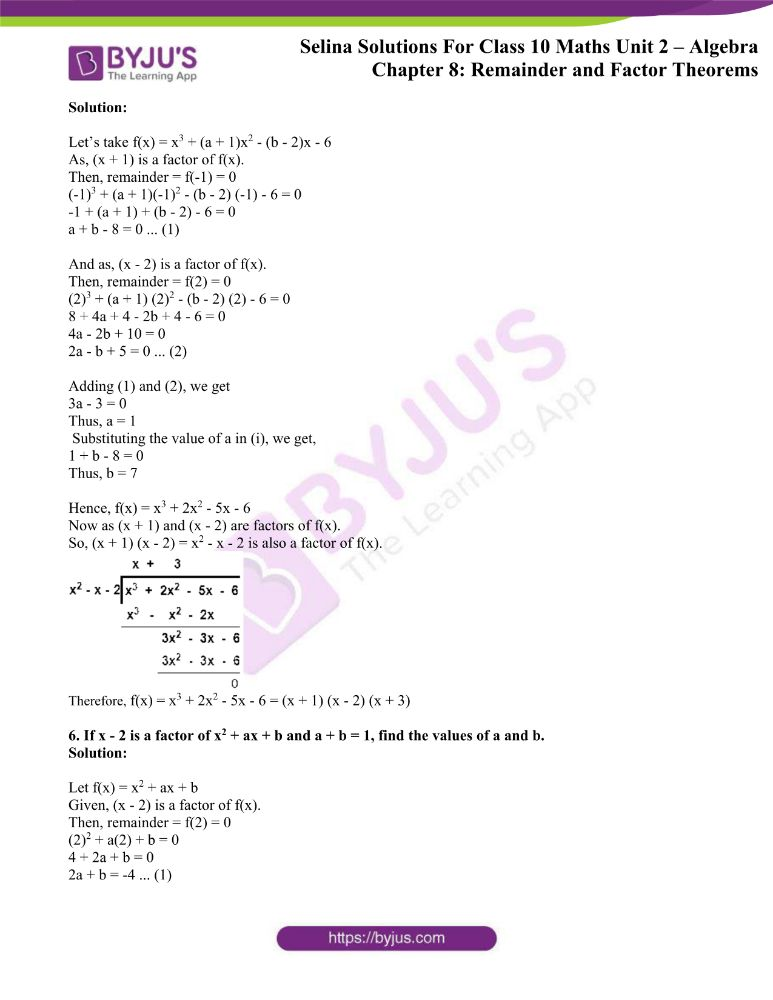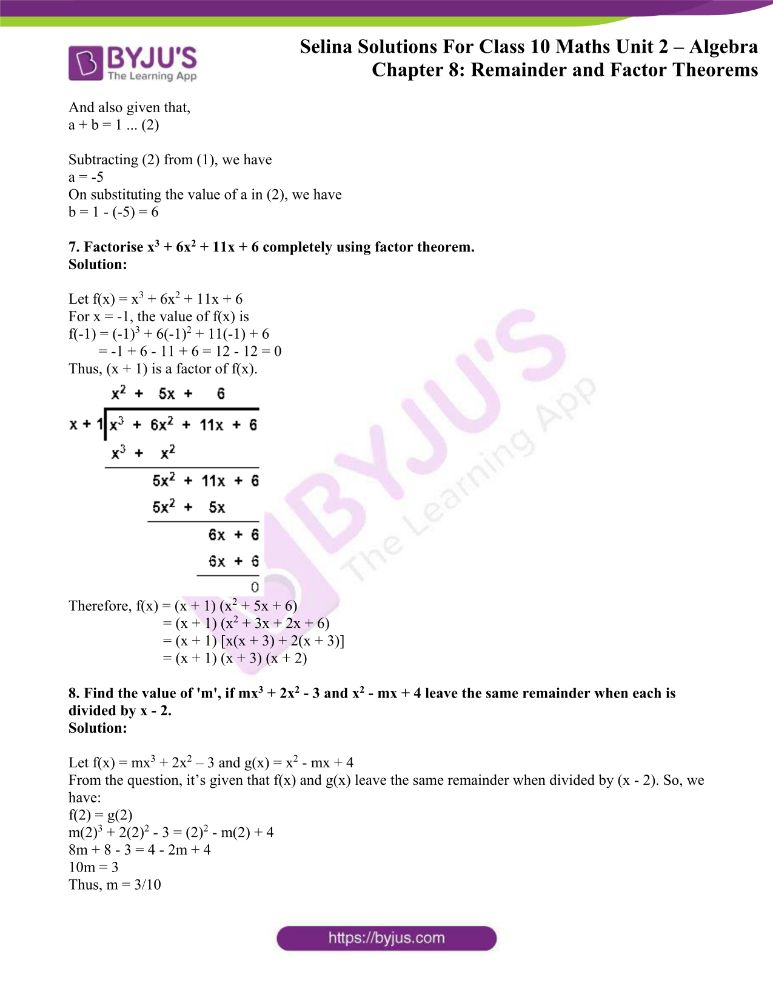### Access other exercises of Selina Solutions Concise Maths Class 10 Chapter 8 Remainder and Factor Theorems

Exercise 8(A) Solutions

Exercise 8(B) Solutions

### Access Selina Solutions Concise Maths Class 10 Chapter 8 Remainder and Factor Theorems Exercise 8(C)

1. Show that (x – 1) is a factor of x3 – 7x2 + 14x – 8. Hence, completely factorise the given expression.

Solution:

Let f(x) = x3 – 7x2 + 14x – 8

Then, for x = 1

f(1) = (1)3 – 7(1)2 + 14(1) – 8 = 1 – 7 + 14 – 8 = 0

Thus, (x – 1) is a factor of f(x).

Now, performing long division we have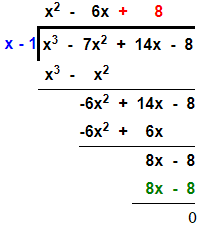Hence, f(x) = (x – 1) (x2 – 6x + 8)

= (x – 1) (x2 – 4x – 2x + 8)

= (x – 1) [x(x – 4) -2(x – 4)]

= (x – 1) (x – 4) (x – 2)

2. Using Remainder Theorem, factorise:

x3 + 10x2 – 37x + 26 completely.

Solution:

Let f(x) = x3 + 10x2 – 37x + 26

From remainder theorem, we know that

For x = 1, the value of f(x) is the remainder

f(1) = (1)3 + 10(1)2 – 37(1) + 26 = 1 + 10 – 37 + 26 = 0

As f(1) = 0, x – 1 is a factor of f(x).

Now, performing long division we have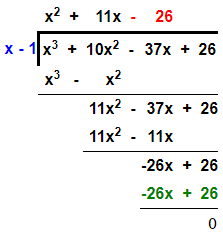Thus, f(x) = (x – 1) (x2 + 11x – 26)

= (x – 1) (x2 + 13x – 2x – 26)

= (x – 1) [x(x + 13) – 2(x + 13)]

= (x – 1) (x + 13) (x – 2)

3. When x3 + 3x2 – mx + 4 is divided by x – 2, the remainder is m + 3. Find the value of m.

Solution:

Let f(x) = x3 + 3x2 – mx + 4

From the question, we have

f(2) = m + 3

(2)3 + 3(2)2 – m(2) + 4 = m + 3

8 + 12 – 2m + 4 = m + 3

24 – 3 = m + 2m

3m = 21

Thus, m = 7

4. What should be subtracted from 3x3 – 8x2 + 4x – 3, so that the resulting expression has x + 2 as a factor?

Solution:

Let’s assume the required number to be k.

And let f(x) = 3x3 – 8x2 + 4x – 3 – k

From the question, we have

f(-2) = 0

3(-2)3 – 8(-2)2 + 4(-2) – 3 – k = 0

-24 – 32 – 8 – 3 – k = 0

-67 – k = 0

k = -67

Therefore, the required number is -67.

5. If (x + 1) and (x – 2) are factors of x3 + (a + 1)x2 – (b – 2)x – 6, find the values of a and b. And then, factorise the given expression completely.

Solution:

Let’s take f(x) = x3 + (a + 1)x2 – (b – 2)x – 6

As, (x + 1) is a factor of f(x).

Then, remainder = f(-1) = 0

(-1)3 + (a + 1)(-1)2 – (b – 2) (-1) – 6 = 0

-1 + (a + 1) + (b – 2) – 6 = 0

a + b – 8 = 0 … (1)

And as, (x – 2) is a factor of f(x).

Then, remainder = f(2) = 0

(2)3 + (a + 1) (2)2 – (b – 2) (2) – 6 = 0

8 + 4a + 4 – 2b + 4 – 6 = 0

4a – 2b + 10 = 0

2a – b + 5 = 0 … (2)

Adding (1) and (2), we get

3a – 3 = 0

Thus, a = 1

Substituting the value of a in (i), we get,

1 + b – 8 = 0

Thus, b = 7

Hence, f(x) = x3 + 2x2 – 5x – 6

Now as (x + 1) and (x – 2) are factors of f(x).

So, (x + 1) (x – 2) = x2 – x – 2 is also a factor of f(x).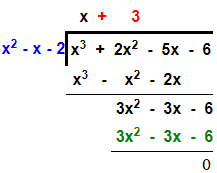Therefore, f(x) = x3 + 2x2 – 5x – 6 = (x + 1) (x – 2) (x + 3)

6. If x – 2 is a factor of x2 + ax + b and a + b = 1, find the values of a and b.

Solution:

Let f(x) = x2 + ax + b

Given, (x – 2) is a factor of f(x).

Then, remainder = f(2) = 0

(2)2 + a(2) + b = 0

4 + 2a + b = 0

2a + b = -4 … (1)

And also given that,

a + b = 1 … (2)

Subtracting (2) from (1), we have

a = -5

On substituting the value of a in (2), we have

b = 1 – (-5) = 6

7. Factorise x3 + 6x2 + 11x + 6 completely using factor theorem.

Solution:

Let f(x) = x3 + 6x2 + 11x + 6

For x = -1, the value of f(x) is

f(-1) = (-1)3 + 6(-1)2 + 11(-1) + 6

= -1 + 6 – 11 + 6 = 12 – 12 = 0

Thus, (x + 1) is a factor of f(x).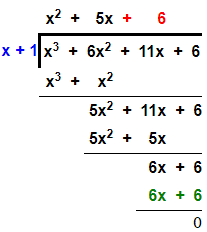Therefore, f(x) = (x + 1) (x2 + 5x + 6)

= (x + 1) (x2 + 3x + 2x + 6)

= (x + 1) [x(x + 3) + 2(x + 3)]

= (x + 1) (x + 3) (x + 2)

8. Find the value of ‘m’, if mx3 + 2x2 – 3 and x2 – mx + 4 leave the same remainder when each is divided by x – 2.

Solution:

Let f(x) = mx3 + 2x2 – 3 and g(x) = x2 – mx + 4

From the question, it’s given that f(x) and g(x) leave the same remainder when divided by (x – 2). So, we have:

f(2) = g(2)

m(2)3 + 2(2)2 – 3 = (2)2 – m(2) + 4

8m + 8 – 3 = 4 – 2m + 4

10m = 3

Thus, m = 3/10